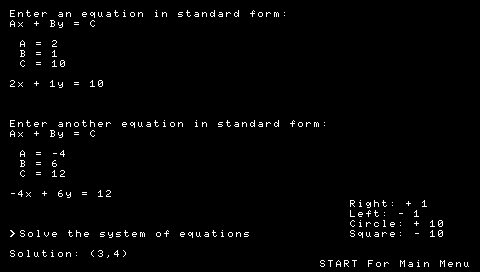Here is a simple application that can compute 4 (well actually, 5) different algebraic values. They are:1) Slope of a line in standard form
2) Y-intercept of a line in standard form
3) Determinant of a 2×2 matrix
4) Determinant of a 3×3 matrix
5) Solution of a system of 2 equations with 2 variables each*

* In other words, it finds the intersection of two lines in standard form.